## GRASS IS GREENER

### AREA OF A RECTANGLE

Students will use the row and column structure to determine the area of a rectangle through the context of laying sod in order to generalize a formula.

## Intentionality & Unit Overview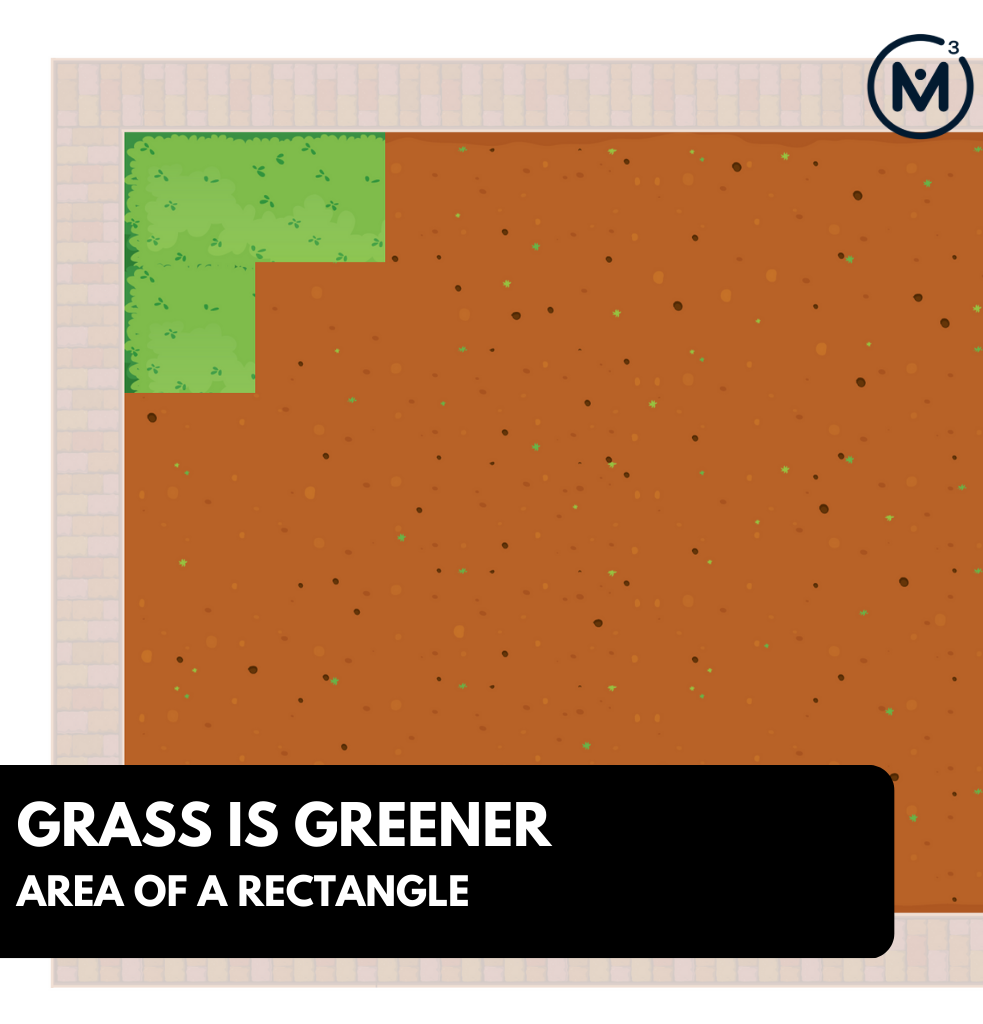### Access each lesson from this unit using the navigation links below

Students will explore how to measure the area of a rectangle through the context of laying sod. The pieces of sod represent the square units. The purpose of today’s lesson is to emerge the array model as a tool to calculate the area of a rectangle. Throughout this unit, students will work towards conceptually understanding the formula for the area of a rectangle.

## Intentionality…

In this task, students will be asked to cover a backyard in square pieces of sod. The task will encourage students to use rows and columns to completely cover the area. The intention of today’s task is for students to make a connection between the array model, and the measurement of area. Through the consolidation, students will formalize the idea that area can be measured by multiplying the length and the width of the rectangle. Some of the big ideas revealed through this task include:

• To measure the area of a rectangle, it must be completely covered by units of area (square units), without gaps or overlaps;
• The alignment of square units produces the rows and columns of an array, with the same number of units in each row;
• The array replaces the need to count individual units and makes it possible to calculate an area;
• Both the number of units in each row and the number of units in a column can be determined from the length of the rectangle’s sides.

## What Do You Notice? What Do You Wonder?

Show students this video:

What do you notice?

What do you wonder?

Give students 60 seconds (or more) to do a rapid write on a piece of paper.

Then, ask students to share with their neighbours for another 60 seconds.

Finally, allow students to share with the entire group.

Some of the noticing and wondering that came up in a class recently included:

• I notice a rectangle.
• I notice small green squares being placed.
• I wonder if this is a yard.
• I wonder if the green is grass.
• I wonder how many pieces of grass are placed in the yard.

At this point, you can answer any notices and wonders that you can cross off the list right away.

For example:

• This is a backyard.
• The backyard is being covered in sod.
• Sod is pre-cut sections of grass that is used to cover a yard quickly instead of planting grass seed and having to wait for it to grow.

## Estimation: Prompt

Next, share the following visual prompt briefly and ask the following question:

Each piece of sod (grass) placed on the ground in the backyard measures 1 unit by 1 unit. We call this a square unit. Estimate how many square units will cover the entire area of this backyard?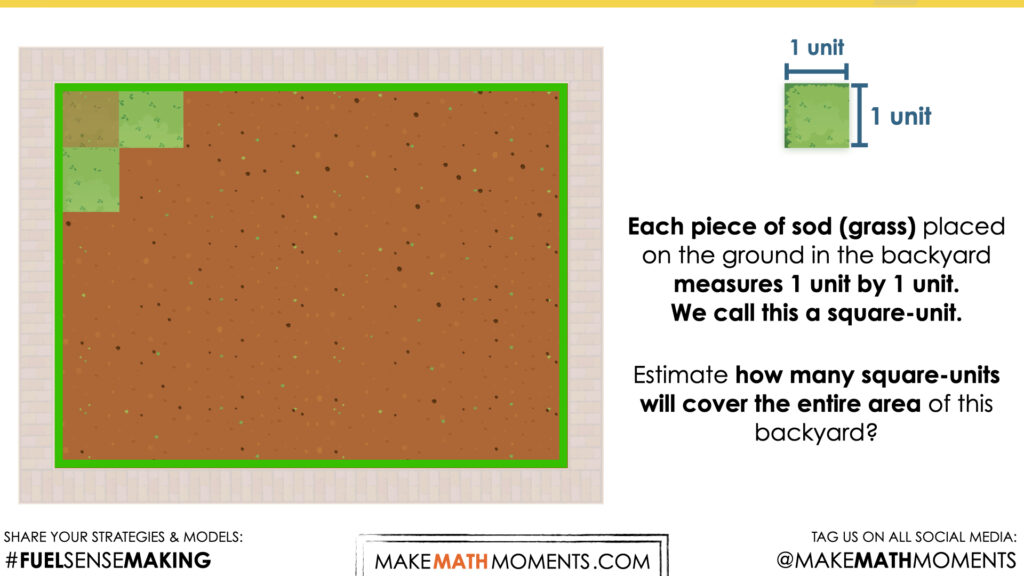We can now ask students to make an estimate (not a guess) as we want them to be as strategic as they can possibly be. This will force them to use reasoning to try and come up with a reasonable measurement.

As students share their estimates, consider drawing a number line to represent the range of estimates and lead a discussion about how confident the group is that the actual number of pieces of sod will land within the range.

## While Students Are Estimating…

Monitor student thinking by circulating around the room and listening to the mathematical discourse. Encourage students to use precise mathematical language and listen for student understanding of how to determine the area of a rectangle. Are students visualizing an array model made of rows and columns?

Allow students to share their estimates with neighbours first, then with the class. Write down their estimates on the chalkboard/whiteboard/chart paper so students feel their voices are being heard and so they feel they have a stake in solving this problem.

Some students might visualize how many square units will fill one row, and how many square units are needed to fill a column. For example, they might say, “two units does not fill half the width of the yard, so they will need more than four pieces down”.

## Crafting A Productive Struggle: Prompt

Since you have already taken some time to set the context for this problem and student curiosity is already sparked, we have them in a perfect spot to help push their thinking further and fuel sense making.

Share the following silent animation that fills in the number of square units needed to complete one row and one column of the backyard.

You can verbally share the following prompt with students:

How many square pieces of sod will cover the entire backyard? Use a model to justify your answer.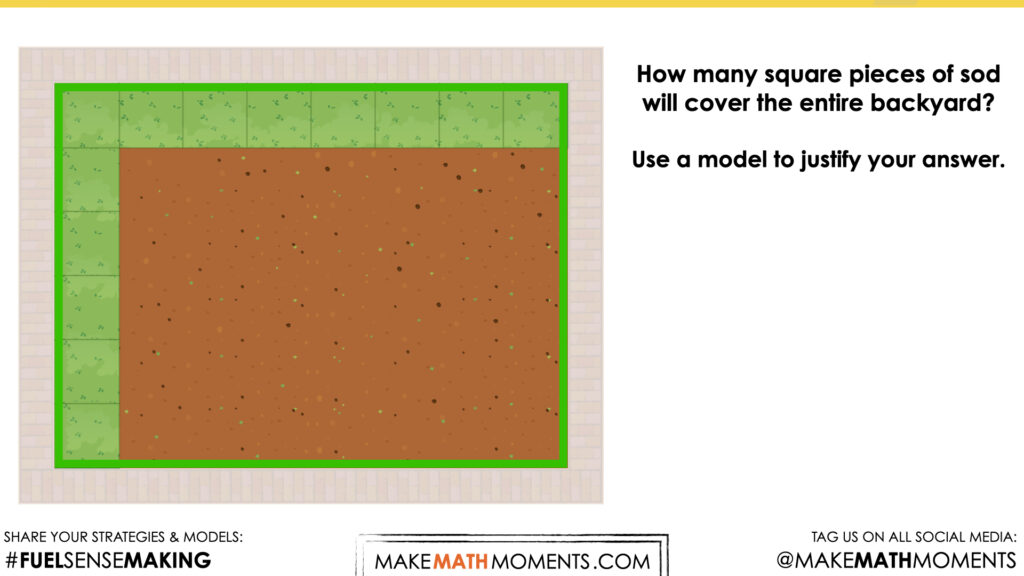## While Students Are Productively Struggling…

Monitor student thinking by circulating around the room and listening to the mathematical discourse.

Select and sequence some of the student solution strategies and ask a student from the selected groups to share with the class from:

• most accessible to least accessible solution strategies and representations;
• most common/frequent to least common/frequent strategies and representations; or,
• choose another approach to selecting and sequencing student work.

The strategies you might see students use include:

• Counting the square pieces of sod;
• Skip counting;
• Using multiplicative thinking.

Have students share their reasoning for determining the area of the yard in square units of sod.

Discuss their thinking during your consolidation to build off of their current prior knowledge and understanding rather than “fixing” or “funnelling” student thinking to a representation and justification that does not connect to their understanding.

## Student Approach #1: Counting with a Concrete Model

I started by using the square tiles to show what we saw in the video, 8 squares across and 6 squares down. I filled in the rectangle completely with more tiles, then I counted. I counted 48 squares altogether.

## Student Approach #2: Skip Counting with a Grid Array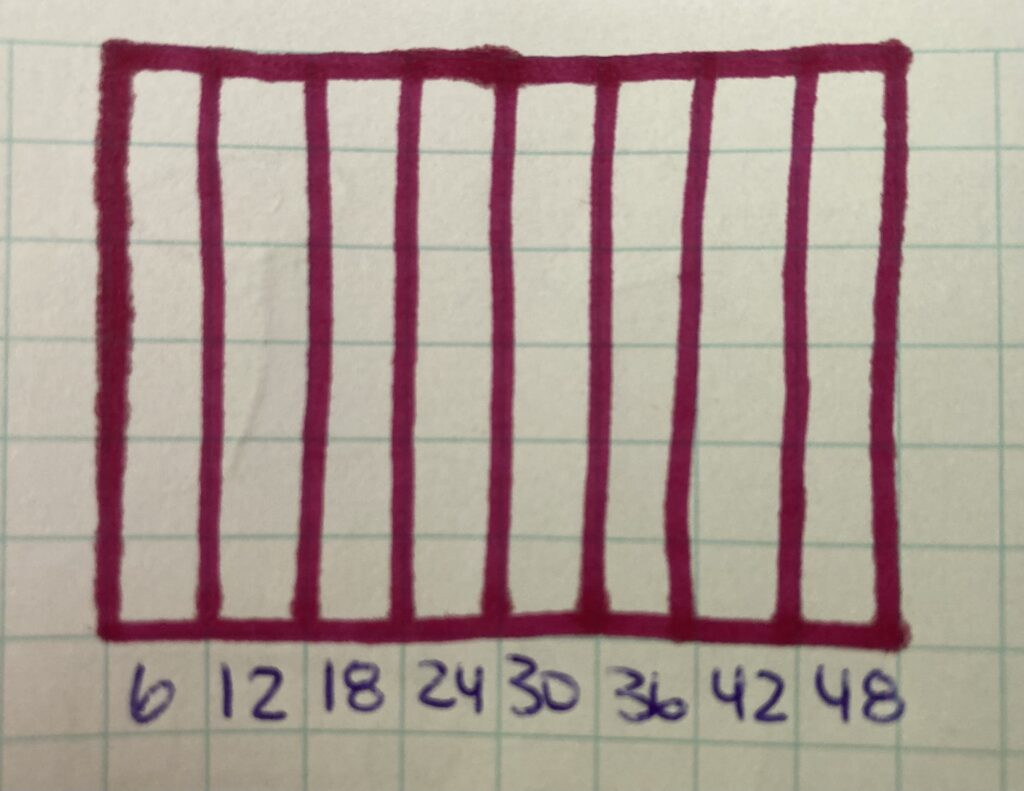I drew a rectangle on grid paper that is 8 squares across and 6 squares down. I just need to count up the squares. I decided to count 6 squares at a time. I skip counted by 6 to 48. There are 48 squares in the backyard.

## Student Approach #3: Using Partial Products with an Open-Array

###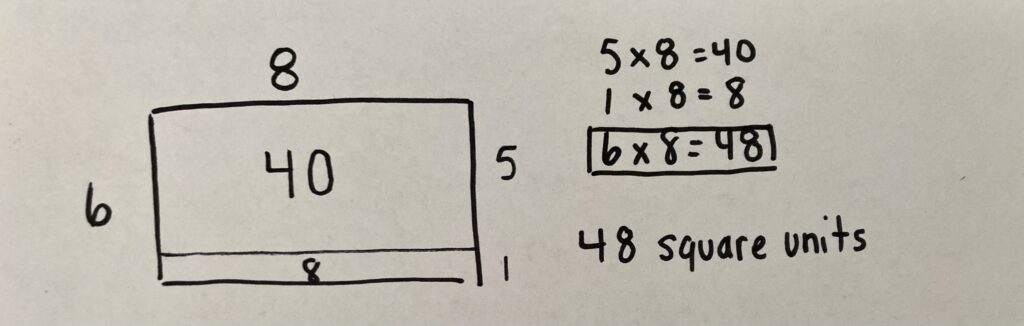I drew a rectangle that is 6 by 8. I know that 5 rows of 8 is 40. So one more row of 8 is 48. There are 48 pieces of sod in the backyard.

## Reveal

Show students different variations of the reveal videos below.

Reveal video #1:

Consider sharing the following screenshots with students: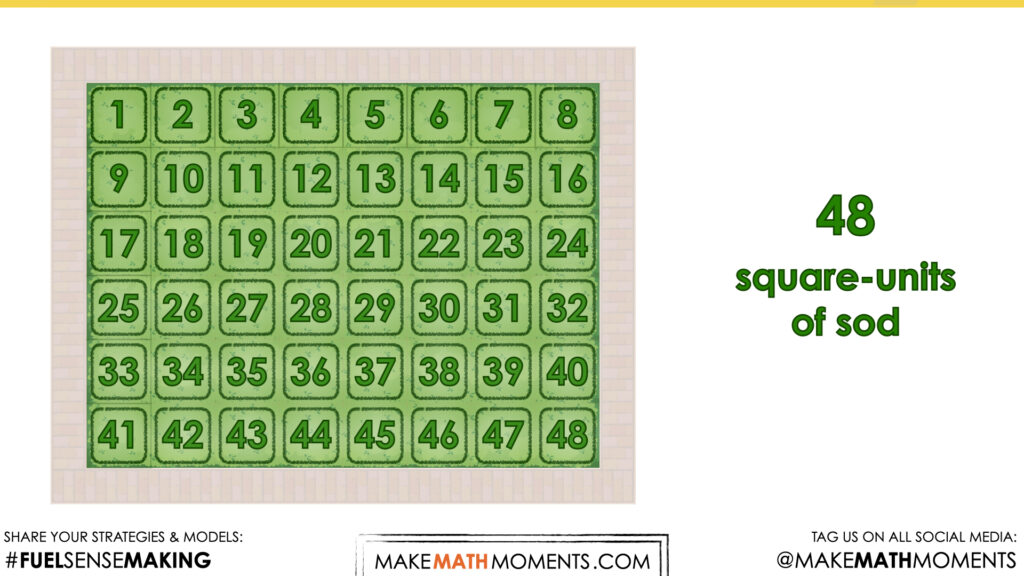Reveal video #2:

Here is a screenshot of the final frame of reveal video #2: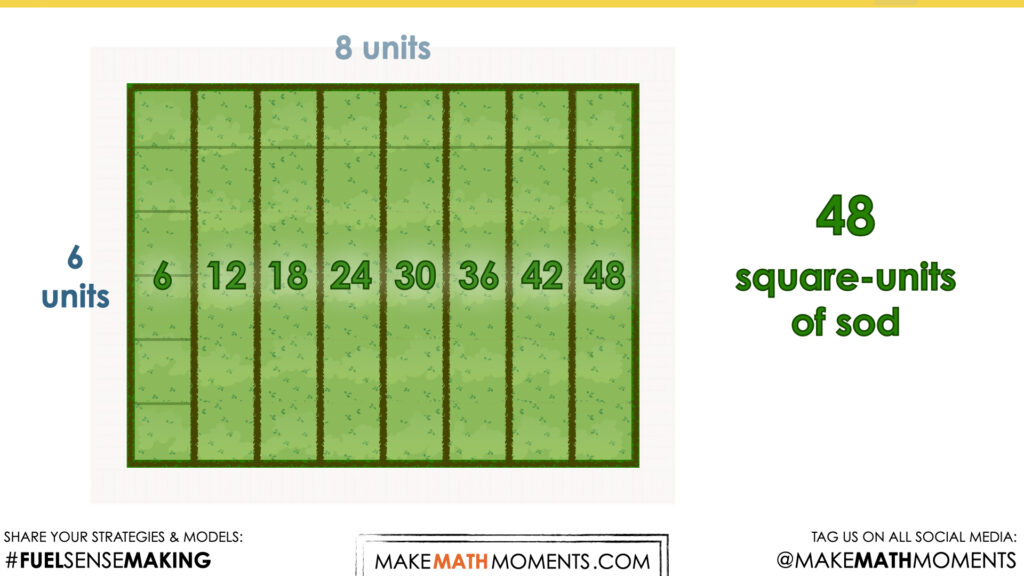Reveal video #3:

Here is a screenshot of the final frame of reveal video #3: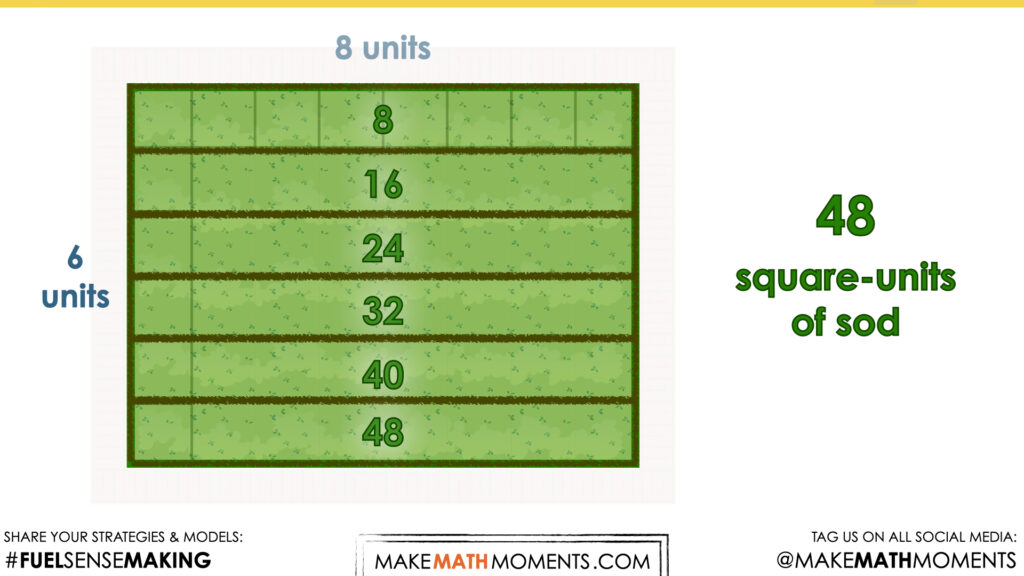Reveal video #4:

Here is a screenshot of the final frame of reveal video #4:Return to the estimates from the beginning of today’s lesson. Celebrate the estimates closest to 48 pieces of sod.

## Consolidation

Leverage student examples as an opportunity to explore each of the following big ideas:

• To measure the area of a rectangle, it must be completely covered by units of area (square units), without gaps or overlaps.
• The alignment of square units produces the rows and columns of an array, with the same number of units in each row.
• The array replaces the need to count individual units and makes it possible to calculate an area.
• Both the number of units in each row and the number of units in a column can be determined from the length of the rectangle’s sides.

Through the use of student examples, land on the measurement of 48 square units of sod. It is important to explicitly state that this is an example of measuring area.

What is area?

Give them an opportunity to turn and talk before sharing ideas with the whole group. Help students land on the following definition:

• Area is the size of a surface measured in square units.
• Area measures the amount of space occupied within the shape.

Give them an opportunity to turn and talk before sharing ideas with the whole group. Help students land on the following idea:

• Thinking about a row or a column as “a group that is repeated” (unitized) connects the array to multiplication: the base of a rectangle corresponds to the number of squares in a row and the height of a rectangle corresponds to the number of squares in a column.

## Reflect and Consolidation Prompts

Provide students an opportunity to reflect on their learning by offering this consolidation prompt to be completed independently.

### Consolidation Prompt #1:

What is the area of the blue rectangle in square units? Justify your answer.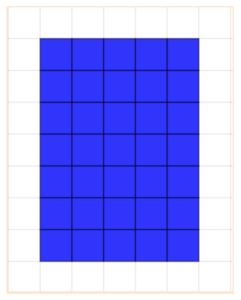### Consolidation Prompt #2:

Jane says that measuring the area is a lot like multiplication. Is this statement true? Justify your answer.

We suggest collecting this reflection as an additional opportunity to engage in the formative assessment process to inform next steps for individual students as well as how the whole class will proceed.

## Educator Discussion Area

Login/Join to access the entire Teacher Guide, downloadable slide decks and printable handouts for this lesson and all problem based units.

## Explore Our 60+ Problem Based Units

This Make Math Moments Lesson was designed to spark curiosity for a multi-day unit of study with built in purposeful practice, number talks and extensions to elicit and emerge strategies and mathematical models.

Dig into our other units of study and view by concept continuum, grade or topic!